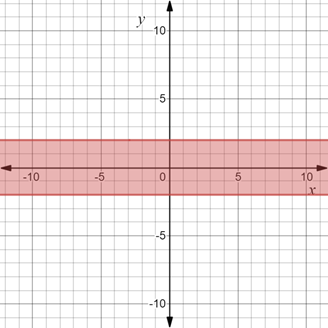# To graph : The region provided by the set { ( x , y ) | | y | ≤ 2 } .### Precalculus: Mathematics for Calcu...

6th Edition
Stewart + 5 others
Publisher: Cengage Learning
ISBN: 9780840068071### Precalculus: Mathematics for Calcu...

6th Edition
Stewart + 5 others
Publisher: Cengage Learning
ISBN: 9780840068071

#### Solutions

Chapter 1.8, Problem 30E
To determine

## To graph: The region provided by the set {(x,y)||y|≤2} .

Expert Solution

### Explanation of Solution

Given information:

The set, {(x,y)||y|2} .

Graph:

The graph of the region provided by the set {(x,y)||y|2} is sketched in the coordinate plane.

Recall that |x|a is equivalent to axa .

Therefore, |y|2 is equivalent to 2y2 .

Construct horizontal lines y=2 and y=2 and shaded the region between it.

The region obtained is provided below,Interpretation:

The region provided by the set {(x,y)||y|2} is shaded region. The dark horizontal line at y=2 and y=2 denote that they are included in the region.The region consists of all those points whose y-coordinate lie between y=2 and y=2 . Therefore, shaded region is the region between horizontal lines y=2 and y=2 and these two horizontal lines are included in the region.

### Have a homework question?

Subscribe to bartleby learn! Ask subject matter experts 30 homework questions each month. Plus, you’ll have access to millions of step-by-step textbook answers!